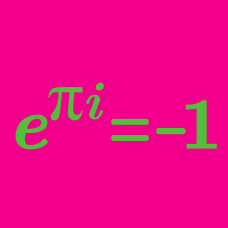Algebra

# De Moivre's Theorem - Roots

Let $z$ be a complex number such that $z = \sqrt{2}(\cos 7 ^{\circ} + i \sin 7 ^{\circ}).$ Then $z^{8}$ can be expressed as $r( \cos \alpha^{\circ} + i \sin \alpha^{\circ})$, where $r$ is a real number and $0 \leq \alpha \leq 90$. What is the value of $r+\alpha ?$

Details and assumptions

$i$ is the imaginary unit, where $i^2=-1$.

The two roots of $f(x) = x^2 + 20x + 200$ can be written in the form $a \pm bi$. What is $b-a,$ assuming $b > 0$?

Details and assumptions

$i$ is defined as the imaginary unit, such that $i^2 = -1$.

Let $z=\cos \alpha^\circ+i\sin \alpha^\circ$ be a complex number such that $0 < \alpha < 20$ and $\displaystyle z^{15}=\frac{1+\sqrt{3}i}{2}.$ What is the value of $\alpha$?

Let $a+bi$ and $c+di$ be the two roots of the quadratic equation $x^2+25=0,$ where $b>d$. What is the value of $ac-bd$?

Details and assumptions

$i$ is the imaginary number that satisfies $i^2 = -1$.

Let $z=\cos \alpha+i\sin \alpha$ be a complex number that satisfies $12 ^\circ < \alpha < 36 ^\circ$ and $z^{30}=1.$ If $z$ can be expressed in polar form as $z=\cos \alpha^\circ+i\sin \alpha^\circ,$ what is the value of $\alpha$ (in degrees)?

×